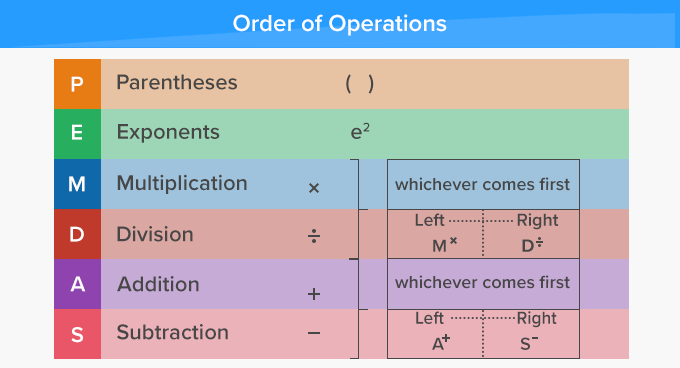# Order Of Operations - Definition with Examples

The Complete K-5 Math Learning Program Built for Your Child

• 30 Million Kids

Loved by kids and parent worldwide

• 50,000 Schools

Trusted by teachers across schools

• Comprehensive Curriculum

Aligned to Common Core

## What is Order of Operations?

In math, order of operations are the rules that state the sequence in which the multiple operations in an expression should be solved.

A way to remember the order of the operations is PEMDAS, where in each letter stands for a mathematical operation.

 P Parentheses E Exponent M Multiplication D Division A Addition S Subtraction

The PEMDAS rules that state the order in which the operations in an expression should be solved, are:
1. Parentheses - They take precedence over all other operators. The first step is to solve all the operations within the parentheses. Work out all groupings from inside to out. (Whatever is in parentheses is a grouping)
2. Exponents - Work out all the exponential expressions.
3. Multiplication and Division - Next, moving from left to right, multiply and/or divide whichever comes first.
4. Addition and Subtraction - Lastly, moving from left to right, add and/or subtract whichever comes first.## Why Follow Order of Operations?

Follow the rules of the order of operations to solve expressions so that everyone arrives at the same answer.

Here’s an example of how we can get different answers if the correct order of operations is NOT followed.
 Expression solved from Left to Right Expression solved using Order of Operations (PEMDAS) 6 x 3 + 4 x ( 9 ÷ 3 ) 6 X 3 + 4 x ( 9 ÷ 3 ) 18 + 4 x ( 9 ÷ 3 ) 22 x ( 9 ÷ 3 ) 198 ÷ 3 = 66     ✘ 6 x 3 + 4 x ( 9 ÷ 3 ) 6 X 3 + 4 x ( 9 ÷ 3 ) → P 6 X 3 + 4 x 3 → M 18 + 4 x 3 → M 18 + 12 → A = 30     ✔

 Fun Facts A popular mnemonic used to remember the order of operations -PEMDAS is ‘Please Excuse My Dear Aunt Sally’.

###Let's sing!

It's all really about the operations,
Solve in order, else there'll be tensions.
Start by opening the Parentheses.
Jump up with the Exponents.
Cube or Square - it's all very fair!
Next, Multiply or Divide - jus' go left to right.
Add or Subtract come last but they’re easy.
finally, it's as simple as A B C D!

###Let's do it!

Instead of handing out practice worksheets to your child, form word problems from real life situations. This will help them write and solve expressions and use the order of operations to simplify expressions in pre-algebra and algebra.
For instance, take your child out for shopping. Ask them to pick out 2 dozen eggs, 3 packets of hot dog buns, 2 packets of candy and 2 boxes of cereal. Then, ask them to put one box of cereal back. Now, ask your child the number of eggs in a dozen, number of buns in a packet, number of candies in a packet and calculate the total number of items bought. Ask them to form an expression and use the order of operations to find the answer.

###Related math vocabulary

Won Numerous Awards & Honors